Water-Jug Problem

Water Jug Problem:

Problem: You are given two jugs, a 4-gallon one and a 3-gallon one.Neither has any measuring mark on it.There is a pump that can be used to fill the jugs with water.How can you get exactly 2 gallons of water into the 4-gallon jug.

Solution:
The state space for this problem can be described as the set of ordered pairs of integers (x,y)
Where,
X represents the quantity of  water in the 4-gallon jug  X= 0,1,2,3,4
Y represents the quantity of water in 3-gallon jug Y=0,1,2,3
Start State: (0,0)
Goal State: (2,0)
Generate production rules for the water jug problem
Production Rules:
 Rule State Process 1 (X,Y | X<4) (4,Y) {Fill 4-gallon jug} 2 (X,Y |Y<3) (X,3) {Fill 3-gallon jug} 3 (X,Y |X>0) (0,Y) {Empty 4-gallon jug} 4 (X,Y | Y>0) (X,0) {Empty 3-gallon jug} 5 (X,Y | X+Y>=4 ^ Y>0) (4,Y-(4-X)) {Pour water from 3-gallon jug into 4-gallon jug until 4-gallon jug is full} 6 (X,Y | X+Y>=3 ^X>0) (X-(3-Y),3) {Pour water from 4-gallon jug into 3-gallon jug until 3-gallon jug is full} 7 (X,Y | X+Y<=4 ^Y>0) (X+Y,0) {Pour all water from 3-gallon jug into 4-gallon jug} 8 (X,Y | X+Y <=3^ X>0) (0,X+Y) {Pour all water from 4-gallon jug into 3-gallon jug} 9 (0,2) (2,0) {Pour 2 gallon water from 3 gallon jug into 4 gallon jug}

Initialization:
Start State: (0,0)
Apply Rule 2:
 (X,Y | Y<3)    -> (X,3) {Fill 3-gallon jug}
Now the state is (X,3)

Iteration 1:
Current State: (X,3)
Apply Rule 7:
 (X,Y | X+Y<=4 ^Y>0) (X+Y,0) {Pour all water from 3-gallon jug into 4-gallon jug}
Now the state is (3,0)

Iteration 2:
Current State : (3,0)
Apply Rule 2:
 (X,Y | Y<3)    -> (3,3) {Fill 3-gallon jug}
Now the state is (3,3)

Iteration 3:
Current State:(3,3)
Apply Rule 5:
 (X,Y | X+Y>=4 ^ Y>0) (4,Y-(4-X)) {Pour water from 3-gallon jug into 4-gallon jug until 4-gallon jug is full}
Now the state is (4,2)

Iteration 4:
Current State : (4,2)
Apply Rule 3:
 (X,Y | X>0) (0,Y) {Empty 4-gallon jug}
Now state is (0,2)

Iteration 5:
Current State : (0,2)
Apply Rule 9:
 (0,2) (2,0) {Pour 2 gallon water from 3 gallon jug into 4 gallon jug}
Now the state is (2,0)

Goal Achieved.

State Space Tree:

1.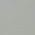2.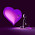nice sir
thanx

3.4.5.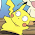6.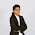Thanks for sharing information about Artificial Intelligence.
Artificial Intelligence Solutions

7.Impressive!Thanks for the post
Artificial intelligence Solutions

8.Can u do it for 5 gallon jug to 2 gallon jug with exactly one gallon of water where 2 gallon jug is empty

1.(0,0) //initial state
(0,2) //rule 2
(2,0) //rule 7
(2,2) //rule 2
(4,0) //rule 7
(4,2) //rule 2
(5,1) //rule 5
(0,1) //rule 3
(1,0) //rule 7 and goal state

9.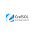Thank You For Sharing this information.
Artificial intelligence Solutions

10.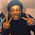11.Thanks for information.
Simple Water Jug Problem

12.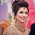Crafsol Technology is the largest artificial intelligence solutions in Pune, India, USA, South Africa, Indonesia, UK, France and Germany provides safer medical procedures, increase in productivity, improving the quality of the physically challenged etc.
Artificial intelligence Solutions

13.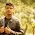14.kids will do (2,0) and (2,3) ..... send me solutions of (2,1) and (2,2)

15.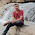I made a java program to do this...

import java.util.*;

public class WaterJugProblem
{

static List SolSet=new ArrayList<>();

public static void main(String args[])
{

System.out.println("------------------WATER JUG PROBLEM SOLVER------------------\n");

Scanner sc=new Scanner(System.in);

System.out.println("Enter capcity of the 2 jugs\n");

int x=sc.nextInt();

int y=sc.nextInt();

System.out.println("Enter target capcity of the 2 jugs\n");

int tx=sc.nextInt();

int ty=sc.nextInt();

sc.close();

FindSolution(0,0,x,y,tx,ty,"",0);

Comparator BestSolution = (p,q)-> p.solCount-q.solCount;

Collections.sort(SolSet,BestSolution);

System.out.println("Total "+SolSet.size()+" possible solutions was found for the problem\n");

if(SolSet.size()!=0)
{
System.out.println("Best Solution is");

System.out.println(SolSet.get(0).sol);
}

System.out.println("Solutions that involve more than 10 steps are ignored to avoid complexity\n");

System.out.println("---------------------------------------------------------Designed By Arpan");

}

static void FindSolution(int x,int y,int xCapacity,int yCapacity,int xTarget,int yTarget,String sol,int count)
{

if(x==xTarget && y==yTarget) // If Goal state is found
{
return;
}

int newX=x,newY=y;
String newSol=sol;

if(count>10) // Too many recursion , Ignore this recursion
return;

//Production Rules

if(x0)
{
newSol=sol;

newX=0;

newY=y;

newSol=newSol+"("+newX+","+newY+")";

newSol=newSol+"Empty first jug\n";

FindSolution(newX,newY,xCapacity,yCapacity,xTarget,yTarget,newSol,count+1);
}

if(y>0)
{
newSol=sol;

newX=x;

newY=0;

newSol=newSol+"("+newX+","+newY+")";

newSol=newSol+"Empty Second jug\n";

FindSolution(newX,newY,xCapacity,yCapacity,xTarget,yTarget,newSol,count+1);
}

if((x+y)>xCapacity && y>0)
{
newSol=sol;

newX=xCapacity;

newY=y-(xCapacity-x);

newSol=newSol+"("+newX+","+newY+")";

newSol=newSol+"Pour water from the second jug into first jug until first jug is full\n";

FindSolution(newX,newY,xCapacity,yCapacity,xTarget,yTarget,newSol,count+1);
}

if((x+y)>yCapacity && x>0)
{
newSol=sol;

newX=x-(yCapacity-y);

newY=yCapacity;

newSol=newSol+"("+newX+","+newY+")";

newSol=newSol+"Pour water from the first jug into second jug until second jug is full\n";

FindSolution(newX,newY,xCapacity,yCapacity,xTarget,yTarget,newSol,count+1);
}

if((x+y)<=xCapacity && y>0)
{
newSol=sol;

newX=x+y;

newY=0;

newSol=newSol+"("+newX+","+newY+")";

newSol=newSol+"Pour all water from second jug into first jug\n";

FindSolution(newX,newY,xCapacity,yCapacity,xTarget,yTarget,newSol,count+1);
}

if((x+y)0)
{
newSol=sol;

newX=0;

newY=y+x;

newSol=newSol+"("+newX+","+newY+")";

newSol=newSol+"Pour all water from first jug into second jug\n";

FindSolution(newX,newY,xCapacity,yCapacity,xTarget,yTarget,newSol,count+1);
}

}
}

class Solution
{
String sol;

int solCount;

Solution(String sol,int solCount)
{
this.sol=sol;
this.solCount=solCount;
}
}

16.17.thanks.....

18.Thanks mr....

19.Isn't rule 9 same as rule 7
Is there a need for rule 9?

20.21.22.